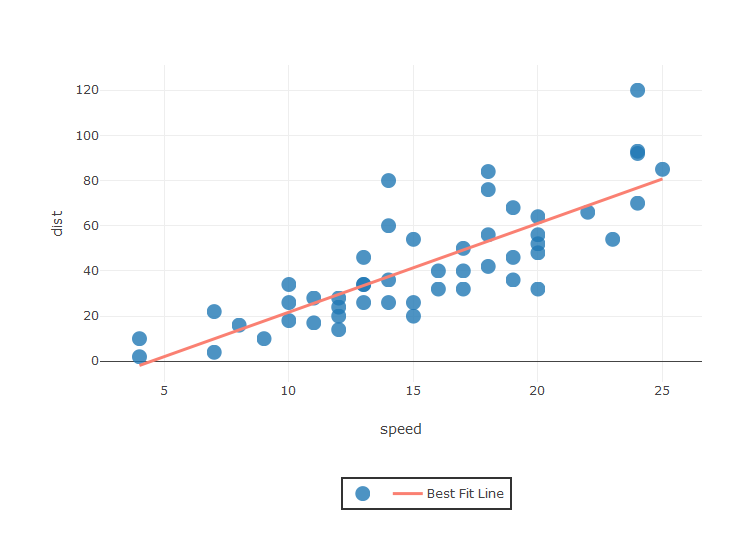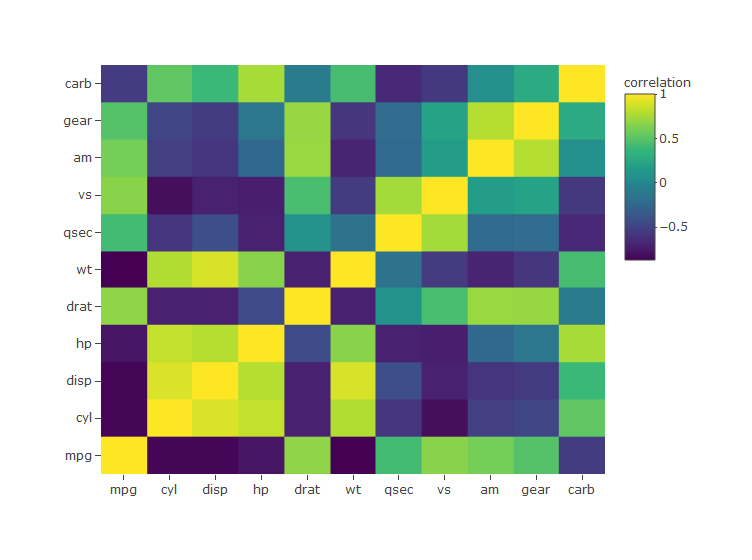## Install Corrly

# install.packages("devtools")
# devtools::install_github("kmaheshKulkarni/corrly")

The package tests the use of htmlwidgets in pkgdown:

## Correlation Scatter Plot

library(plotly)
library(corrly)
spearman<- corr_coef_spearman(variable1= cars$speed, variable2=cars$dist, decimal = 2)
corr_scatterly(data=cars,x=cars$speed,y=cars$dist,corr_coef=spearman,xname="speed",yname="dist")## Correlation Matrix Plot

library(plotly)
library(corrly)
matrixly(data = mtcars)